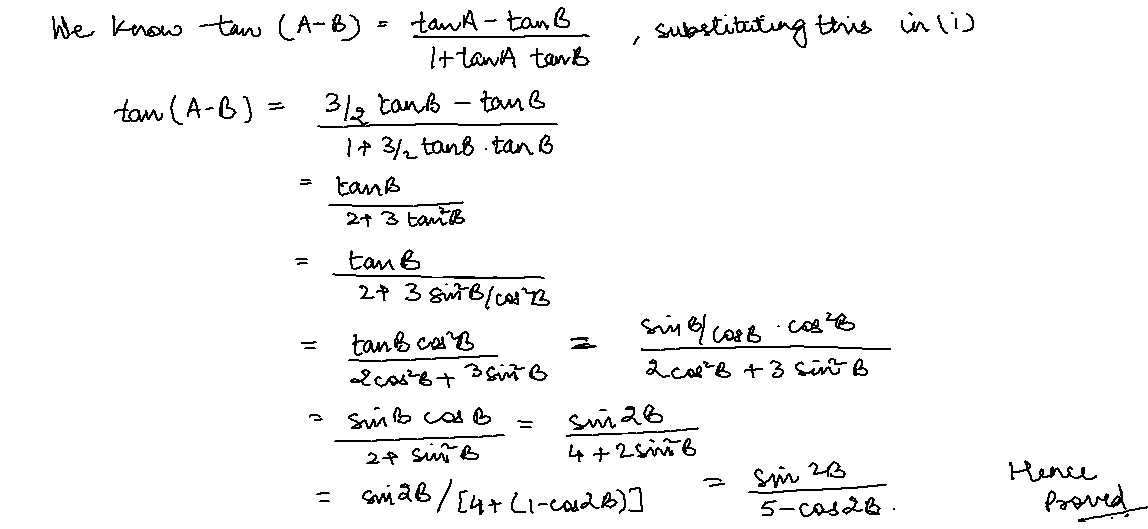×#### Thank you for registering.

One of our academic counsellors will contact you within 1 working day.

Click to Chat

1800-1023-196

+91-120-4616500

CART 0

• 0

MY CART (5)

Use Coupon: CART20 and get 20% off on all online Study Material

ITEM
DETAILS
MRP
DISCOUNT
FINAL PRICE
Total Price: Rs.

There are no items in this cart.
Continue Shopping
```
if 2tanA=3tanB,prove that tan(A-B)=sin2B/5-cos2B

```
5 years agoLatika Leekha
165 Points
```							Hello student,Given that 2 tan A = 3 tan B,So, tan A = 3/2 tan B ….. (i)```
5 years ago
```							Dear Student,Please find below the solution to your problem.If 2tanA=3tanB or tanA=(3/2).tanBProve that tan(A-B)=sin2B/(5-cos2B)LHS.tan(A-B)=(tanA-tanB)/(1+tanA.tanB), put tanA=(3/2).tanB.=[(3/2)tanB-tanB]/[1+(3/2)tan^2B]=[(1/2).tanB]/[(2cos^2B+3sin^2B)/2cos^2B].=[(sinB/2cosB)×2cos^2B]/[2cos^2B+3sin^2B].=[sinBcosB]/(2cos^2B+3sin^2B)=(sin2B)/2.(2cos^2B+3sin^2B=(sin2B)/(4cos^2B+6sin^2B)=(sin2B)/[4(1-sin^2B)+6sin^2B]=(sin2B)/[4–4sin^2B+6sin^2B]=(sin2B)/[4+2sin^2B) , put 2sin^2B=1-cos2B=(sin2B)/[4+1-cos2B]=(sin2B)/(5-cos2B) , Proved.Thanks and Regards
```
3 months ago
Think You Can Provide A Better Answer ?

## Other Related Questions on Trigonometry

View all Questions »### Course Features

• 731 Video Lectures
• Revision Notes
• Previous Year Papers
• Mind Map
• Study Planner
• NCERT Solutions
• Discussion Forum
• Test paper with Video Solution### Course Features

• 31 Video Lectures
• Revision Notes
• Test paper with Video Solution
• Mind Map
• Study Planner
• NCERT Solutions
• Discussion Forum
• Previous Year Exam Questions